# PROPERTIES OF FLUIDReading time: 1 minute

PROPERTIES OF FLUID

#### 1. DENSITY OR MASS DENSITY

Density or mass density of a fluid is defined as the ratio of the mass of a fluid to its volume. Thus mass per unit volume of a fluid is called density.

It is denoted by the symbol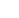(rho). The unit of mass density in SI unit is kg per cubic meter. The density of liquids may be considered as constant while that of gases changes with the variation of pressure and temperature.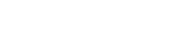The value of density of water is 1gm per cubic centimeter or 1000 kg per cubic meter.

#### 2. SPECIFIC WEIGHT AND WEIGHT DENSITY

Specific weight or weight density of a fluid is the ratio between the weight of a fluid to its volume. Thus weight per unit volume of a fluid is called weight density and it is denoted by the symbol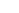.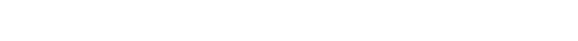The value of specific weight of specific density () of water is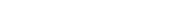#### 3. SPECIFIC VOLUME

Specific volume of a fluid is defined as the volume of a fluid occupied by a unit mass or volume per unit mass of a fluid.

Specific volume =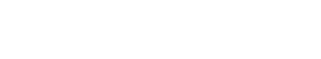Thus, specific volume is the reciprocal of mass density. It is expressed as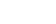. It is commonly applied to gases.

#### 4. SPECIFIC GRAVITY

Specific gravity is defined as the ratio of the weight density (or density) of a fluid to the weight density (or density) of a standard fluid. For liquids, the standard fluid is taken as water and for gases, the standard fluid is taken as air. Specific gravity is also called relative density. It is a dimensionless quantity and is denoted by the symbol S.

S (for liquids) =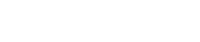S (for gases) =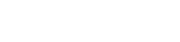Thus, weight density of a liquid = S x weight density of water = S xThe density of liquid = S x Density of water = S x 1000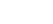.

If the specific gravity of a fluid is known, then the density of the liquid will be equal to specific gravity of fluid multiplied by the density of water. For example, the specific gravity of mercury is 13.6. Hence density of mercury = 13.6 x 1000.

#### 5. VISCOSITY OF LIQUID:

Viscosity is defined as the property of a fluid which offers resistance to the movement of one layer of fluid over another adjacent layer of fluid. When two layers of a fluid, a distance apart move over one other at different velocities, the viscosity together with relative velocity causes a shear stress acting between the fluid layers.

The top layer causes a shear stress on the adjacent layer while the lower layer causes a shear stress on the top layer. This shear stress is proportional to the rate of change of velocity. It is denoted by the symbol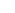.Where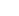(mu) is the constant of proportionality and is known as the coefficient of dynamic viscosity or only viscosity,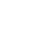represents the rate of shear strain or rate of shear deformation or velocity gradient.The viscosity is also defined as the shear stress required to produce unit rate of shear strain.

Units of viscosity:

In MKS system, unit of viscosity =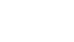CGS unit of viscosity (also called Poise) =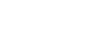SI unit of viscosity =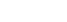=Pa-s

Unit Conversion

Conversion between MKS and CGS system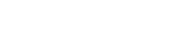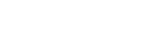1 N = 1000 x 100 dyne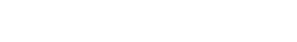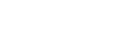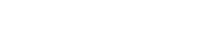#### KINEMATIC VISCOSITY

It is defined as the ratio between the dynamic viscosity and density of fluid. It is denoted by the Greek symbol () called nu. Thus,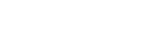In MKS and SI, the unit of kinematic viscosity is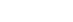while in CGS units, it is written as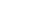. In CGS system, kinematic viscosity is also known as stoke.

One stoke =1Newton’s Law of Viscosity:

It states that the shear stress () on a fluid element layer is directly proportional to the rate of change of shear strain. The constant of proportionality is called the co-efficient of viscosity.Fluids which obey the above relation are known as Newtonian fluids and the fluids which do not obey the above relation are called Non-Newtonian fluids.

#### Variation of Viscosity with temperature:

The viscosity of liquids decreases with the increase in temperature, while the viscosity of gases increases with the increase in temperature.

(i) For liquids: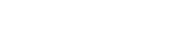Where,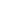= viscosity of liquid at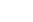in poise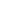= viscosity of liquid at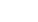in poise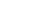are constants for the liquid.

For water,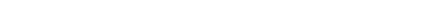(ii) For Gases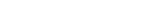For air,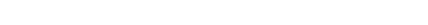#### TYPES OF FLUIDS BASED ON VISCOSITY:

The fluids may be classified into following five types:

1. Ideal fluid
2. Real fluid
3. Newtonian fluid
4. Non-Newtonian fluid
5. Ideal plastic fluidFigure: Type of fluids

1. Ideal fluid:

A fluid which is incompressible and is having no viscosity, is known as ideal fluid. Ideal fluid is only an imaginary fluid as all the fluids which exists have some viscosity.

2. Real Fluids:

A fluid which possesses viscosity is known as real fluid. All the fluids in actual practice are real fluids.

3. Newtonian Fluids:

A real fluid in which the shear stress is directly proportional to rate of shear strain (or velocity gradient).

4. Non-Newtonian Fluid:

A real fluid in which the shear stress is not proportional to the rate of shear strain.

5. Ideal Plastic Fluid:

A fluid in which shear stress is more than the yield value and shear stress is proportional to the rate of shear strain (or velocity gradient).